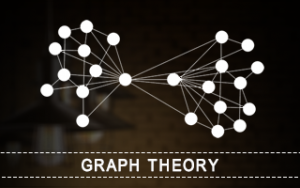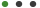Friendship Week Special | Get Additional 10% OFF, use Code "FRIENDS"
Sale Ends In

# Graph TheorySimply Easy Learning Arnab Chakraborty IT & Software, IT Other

• Graph Theory
1 Lectures
• Graph Theory Introduction
3 Lectures
• Graph Theory Fundamentals
7 Lectures
• Graph Basic Properties
2 Lectures
• Types of Graph
12 Lectures
• Trees
6 Lectures
• Graph Coloring
3 Lectures
• Graph Isomorphic
2 Lectures
• Graph Traversibility
7 Lectures
• Graph Representation Techniques
7 Lectures
• Algorithm and Complexity Analysis
8 Lectures
• Algorithm and Classification
13 Lectures
• Graph Theory Algorithm
26 Lectures
• #### Description

This tutorial offers an introduction to the fundamentals of graph theory. It is in a very reader-friendly tutorial style. It covers the types of graphs, their properties, different terminologies, trees, graph traversability, the concepts of graph colouring, different graph representation techniques, concept of algorithms and different graph theory based algorithms.

# Audience

This tutorial has been designed for students who want to learn the basics and algorithms of Graph Theory. Graph Theory has a wide range of applications in engineering and hence, this tutorial will be quite useful for readers who are into Language Processing or Computer Networks, physical sciences and numerous other fields.## Preview Videos

This Course Includes :

7 hours

97 Lectures